New Octave Band Plot for Acoustics Simulation in COMSOL 5.2

January 21, 2016

COMSOL Multiphysics version 5.2 introduced a number of new features and functionality, including an acoustics-specific plot type called the Octave Band plot. This plot type provides you with an easy and flexible way to represent any frequency response, transfer function, sensitivity curve, transmission loss, or insertion loss — all of which are essential plots in many acoustics applications. Let’s learn a bit more about the Octave Band plot, while highlighting its various options and settings.

The Importance of Octaves

When discussing an octave, we are referring to a frequency band in which the upper frequency is twice the lower frequency. Applying this concept results in bands of equal width on a logarithmic frequency axis.

Representing an acoustic response by binning the signal energy in octaves, or fractional octaves, is very common for acoustic and audio engineers. The visualization technique is closely related to specifications in standards and thus the way measurement equipment works (e.g., sound level meters). Physiologically, such representation is motivated by the fact that the human ear itself filters (via auditory filters) and perceives sound on a logarithmic frequency axis. In the same way, the human ear also has a logarithmic sensitivity, hence the use of a decibel scale for levels.

As we will describe next, the new Octave Band plot includes more functionality than simply plotting acoustic responses in octave bands.

The Octave Band Plot, a Simple and Versatile Acoustics-Specific Plot

The new Octave Band plot, available in the Acoustics Module within COMSOL Multiphysics, includes built-in features that are specific to acoustics simulations and can be helpful for representing and analyzing frequency-domain data. The output of the plot is automatically given on the dB scale, with several formatting options. With regards to the data fed to the plot, you can readily pick it up at a point or as an average over a line, surface, or volume.

For the graphs, you have the option to format the results so that they render in either octave bands, 1/3-octave bands, or as a continuous curve. You are also able to easily add weighting to the response curve, with the choice of a Z-, A-, C-, or user-defined weighting. For the input to the plot, you can modify it to represent amplitude (e.g., the absolute value of a pressure); power (e.g., an intensity integrated over a surface); or a general transfer function.

All of these options help to simplify postprocessing considerably. Further, the new plot type makes it much easier to compare results with measured data, which is often given in octave or 1/3-octave bands.

The screenshot below shows the user interface (UI) for the Octave Band plot. In the next section, we will explain its different options and settings in detail.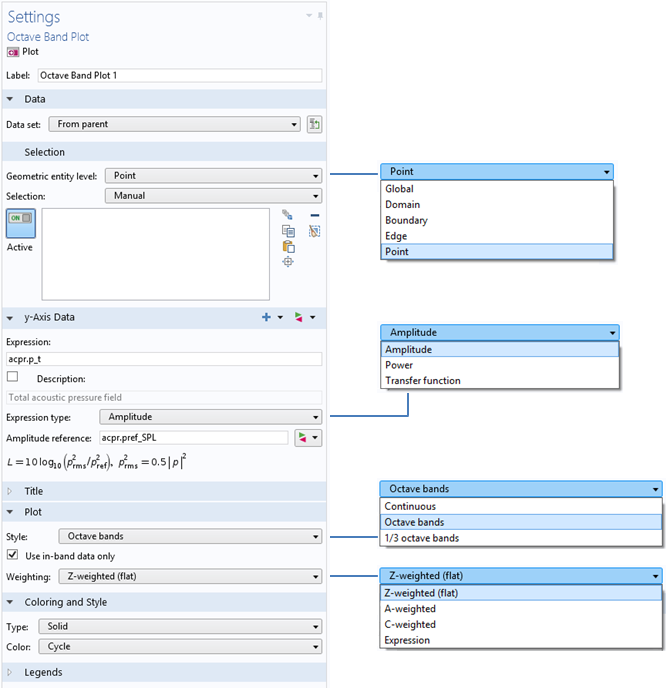The UI for the new Octave Band plot.

An Overview of the Different Options and Settings

Geometric Entity Level

The Geometric entity level selection enables you to select how the input data is picked up from the model via the drop-down menu. The data can be directly taken from a point in the model if, perhaps, a sensitivity is measured at a specific location. By selecting Edge, Boundary, or Domain, the input is automatically averaged. Such an approach is useful when calculating the transmission loss in a muffler system, for instance. Here, the average pressure is easily picked up on the outlet boundary. Thus, it is no longer necessary to set up integration operators (a customary practice in previous versions of COMSOL Multiphysics).

The Geometric entity level selection can also be used to evaluate global quantities. You could, for example, evaluate the pressure in a point outside of the computational domain using the Far-field Calculation feature.

Expression Type

With the Expression type selection, you can decide how the input data in the Octave Band plot is interpreted. There are three available options: Amplitude, Power, and Transfer function.

The default option is Amplitude. When this is selected, the input in the Expression field is treated as a complex amplitude, p, which is most often the pressure in acoustics applications. The entered value is then used to evaluate the level as

L = 10 \log_{10} \left( \frac{p_\textrm{rms}^2}{p_\textrm{ref}^2} \right)

where the rms pressure is calculated as p_\textrm{rms}^2 = 0.5 |p|^2. The reference pressure, p_\textrm{ref}, is entered in the Amplitude reference field and is assumed to be an rms quantity. In most acoustics interfaces, the default value is phys.pref_SPL, which is defined in the sound pressure level settings at the physics interface level. The default value is typically 20 \mu Pa. As an example, when defining a transfer function, the reference amplitude could be set to the rms amplitude, 1/\sqrt{2}\:\textrm{Pa} of an incident plane wave that has a peak amplitude of 1\:\textrm{Pa}, and the input in the Expression field could be the average pressure measured over a surface.

The next option is Power. Here, the input to the plot entered in the Expression field is assumed to be a power, P. The value could be, for example, calculated from an integral of the acoustic intensity over a surface. The level is computed as

L = 10 \log_{10} \left( \frac{P}{P_\textrm{ref}} \right)

where the reference power, P_\textrm{ref}, is entered in the Power reference field. The default is phys.Pref_SWL, which is the reference sound power level of 10^{-12} \: \textrm{W}.

Transfer function is the final option. Any user-defined transfer function, H, and a reference level, L_\textrm{ref}, can be entered in this case. They are used to define the level

L = 10 \log_{10} \left( |H| \right)+L_\textrm{ref}

The input data is used to integrate the power within bands when the results are plotted as octave or 1/3-octave bands, depending on the style that you choose.

Style

Under the Style selection, there is a drop-down menu that gives you different choices for formatting the data. The frequency-domain data can either be plotted in octave bands (the default), in 1/3-octave bands, or as a continuous curve.

The three different plot styles are highlighted in the figure below. Red bars indicate the octave band data, while blue bars represent the 1/3-octave band data. (The formatting of the bars can be changed via the Coloring and Style section.) The color green, meanwhile, depicts the continuous curve. The plot is a modified version of the one from our Loudspeaker Driver tutorial model.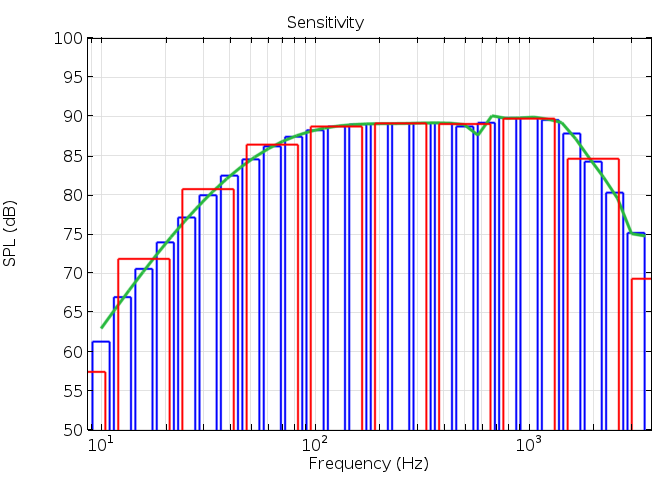A graph depicting the different plot styles.

Weighting

Lastly, using the Weighting selection, you can identify the weighting that should be applied to the data. The choices include:

• Z-weighted (flat): Zero weighting is applied (the default option).
• A-weighted: The IEC 61672-1 standard A-weighting is applied to the data. It is used to account for the perceived loudness of the human ear.
• C-weighted: The IEC 61672-1 standard C-weighting is applied to the data. This option can also account for the perceived loudness of the human ear, usually for very loud levels of noise.
• Expression: A user-defined value or expression is used for the weighting. In the Expression field, an expression that defines the gain as a function of the frequency, freq, is entered. The gain provided in dB is then given as 20·log10(expression).

The two curves in the figure below represent the A- and C-weights applied to a flat 0 dB response. A user-defined linear weighting is given as 10^{-3}\cdot f and is represented in 1/3-octave bands (shown in red).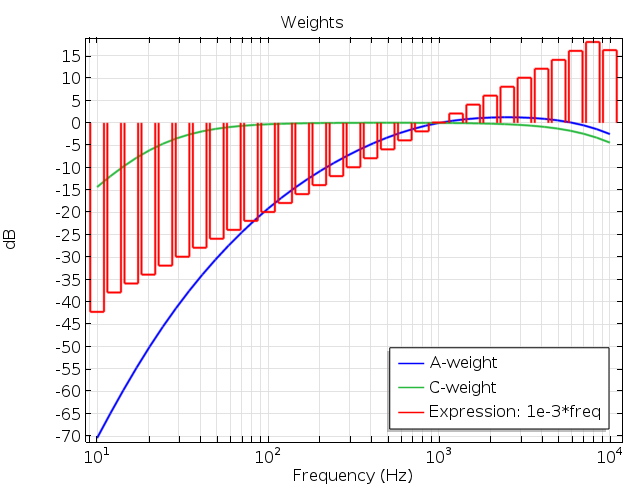A plot showing the different weighting options.

Using an Octave Band Plot: An Absorptive Muffler Example

The Absorptive Muffler tutorial model (located in the Automotive folder within the Application Library) uses the Octave Band plot to depict the transmission loss of a muffler system. In this version of the model, the Transfer function option is selected for the Expression type. The input to the plot is the ratio of the total incident power to the total transmitted power. These elements are calculated by using an integration-coupling operator to take an integral over the inlet and outlet.

There is another way to plot the same transmission loss without having to set up expressions and integration operators. To do so, you set the Geometric entity level to Boundary and select the outlet boundary number 28. In the Expression field, enter p0 (the amplitude of the incident plane wave) and in the Amplitude reference field, enter sqrt(0.5)*acpr.p_t. Remember, it is assumed that the reference is an rms value and, therefore, the square root factor at the front (see the screenshot below). Choosing the 1/3-octave bands plot style and clicking on the Plot button gives the results shown below for the case of a muffler with no liner.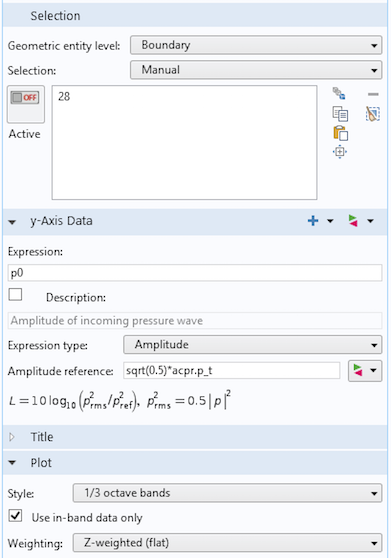Plot settings for the Octave Band plot for the absorptive muffler.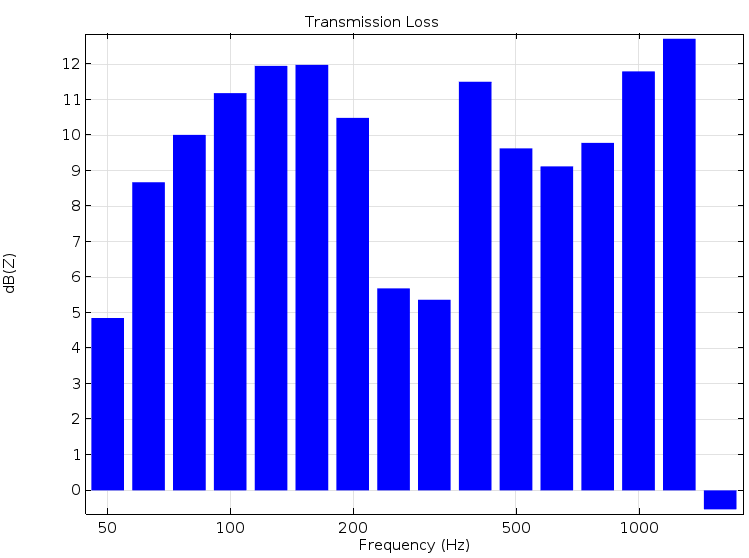Transmission loss plot for the absorptive muffler.

Explore Octave Band Plots with These Resources

In addition to the example referenced above, you can find many other tutorials in the Application Library that utilize the Octave Band plot. We’ve listed a few of those tutorials here, all of which are available for download from our Application Gallery.

Reference

1. IEC 61672-1 Electroacoustics — Sound level meters — Part 1: Specifications.

CatégoriesIan Chou
August 21, 2019

Hi
Why this (where the rms pressure is calculated as prms^2=0.5|P|^2) ?